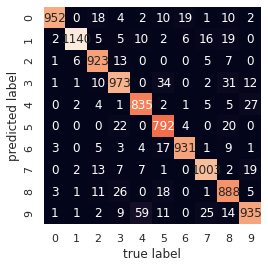Last week on this blog, I presented AdaOpt for R, applied to iris dataset classification. And the week before that, I introduced AdaOpt for Python. AdaOpt is a novel probabilistic classifier, based on a mix of multivariable optimization and a nearest neighbors algorithm. More details about the algorithm can be found in this (short) paper. This week, we are going to train AdaOpt on the popular MNIST handwritten digits dataset without preprocessing, a.k.a neither convolution nor pooling.

Install mlsauce’s AdaOpt from the command line (for R, cf. below):

!pip install git+https://github.com/Techtonique/mlsauce.git --upgrade


Import the packages that will be necessary for the demo:

from time import time
from tqdm import tqdm
import mlsauce as ms
import numpy as np
from sklearn.metrics import classification_report
from sklearn.model_selection import train_test_split
from sklearn.datasets import fetch_openml


Get MNIST handwritten digits data (notice that here, AdaOpt is trained on 5000 digits, and evaluated on 10000):

Z, t = fetch_openml('mnist_784', version=1, return_X_y=True)

print(Z.shape)
print(t.shape)

t_ = np.asarray(t, dtype=int)

np.random.seed(2395)

train_samples = 5000

X_train, X_test, y_train, y_test = train_test_split(
Z, t_, train_size=train_samples, test_size=10000)


Creation of an AdaOpt object:

obj = ms.AdaOpt(**{'eta': 0.13913503573317965, 'gamma': 0.1764634904063013,
'k': np.int(1.2154947405849463),
'learning_rate': 0.6161538857826013,
'n_iterations': np.int(245.55517115592275),
'reg_alpha': 0.29915416038957043,
'reg_lambda': 0.163411853029936,
'row_sample': 0.9477046112286693,
'tolerance': 0.05877163298305207})


Adjusting the AdaOpt object to the training set:

start = time()
obj.fit(X_train, y_train)
print(time()-start)

0.7025153636932373


Obtain the accuracy of AdaOpt on test set:

start = time()
print(obj.score(X_test, y_test))
print(time()-start)

0.9372
9.997464656829834


Classification report including additional error metrics:

preds = obj.predict(X_test)
print(classification_report(preds, y_test))

   precision    recall  f1-score   support

0       0.99      0.94      0.96      1018
1       0.99      0.95      0.97      1205
2       0.93      0.97      0.95       955
3       0.92      0.91      0.91      1064
4       0.91      0.95      0.93       882
5       0.89      0.95      0.92       838
6       0.97      0.96      0.96       974
7       0.95      0.95      0.95      1054
8       0.88      0.93      0.91       953
9       0.93      0.88      0.91      1057

accuracy                           0.94     10000
macro avg       0.94      0.94      0.94     10000
weighted avg       0.94      0.94      0.94     10000


Confusion matrix, true label vs predicted label:

import matplotlib.pyplot as plt
import seaborn as sns; sns.set()
from sklearn.metrics import confusion_matrix

mat = confusion_matrix(y_test, preds)
sns.heatmap(mat.T, square=True, annot=True, fmt='d', cbar=False)
plt.xlabel('true label')
plt.ylabel('predicted label');In R, the syntax is quite similar to what we’ve just demonstrated for Python. After having installed mlsauce, we’d have:

• For the creation of an AdaOpt object:
library(mlsauce)

# create AdaOpt object with default parameters
print(obj$get_params())  • For fitting the AdaOpt object to the training set: # fit AdaOpt to training set obj$fit(X_train, y_train)

• For obtaining the accuracy of AdaOpt on test set:
# obtain accuracy on test set